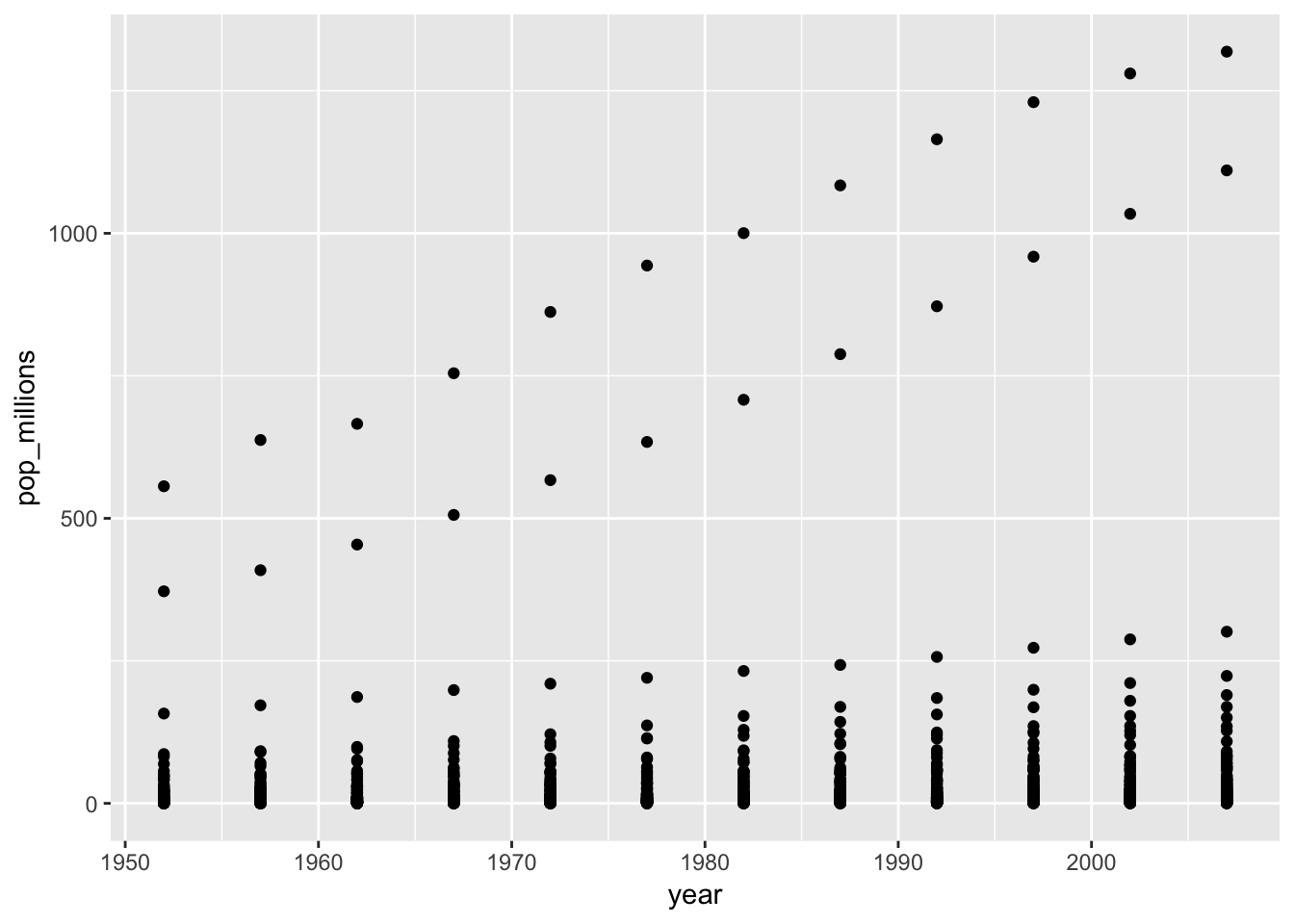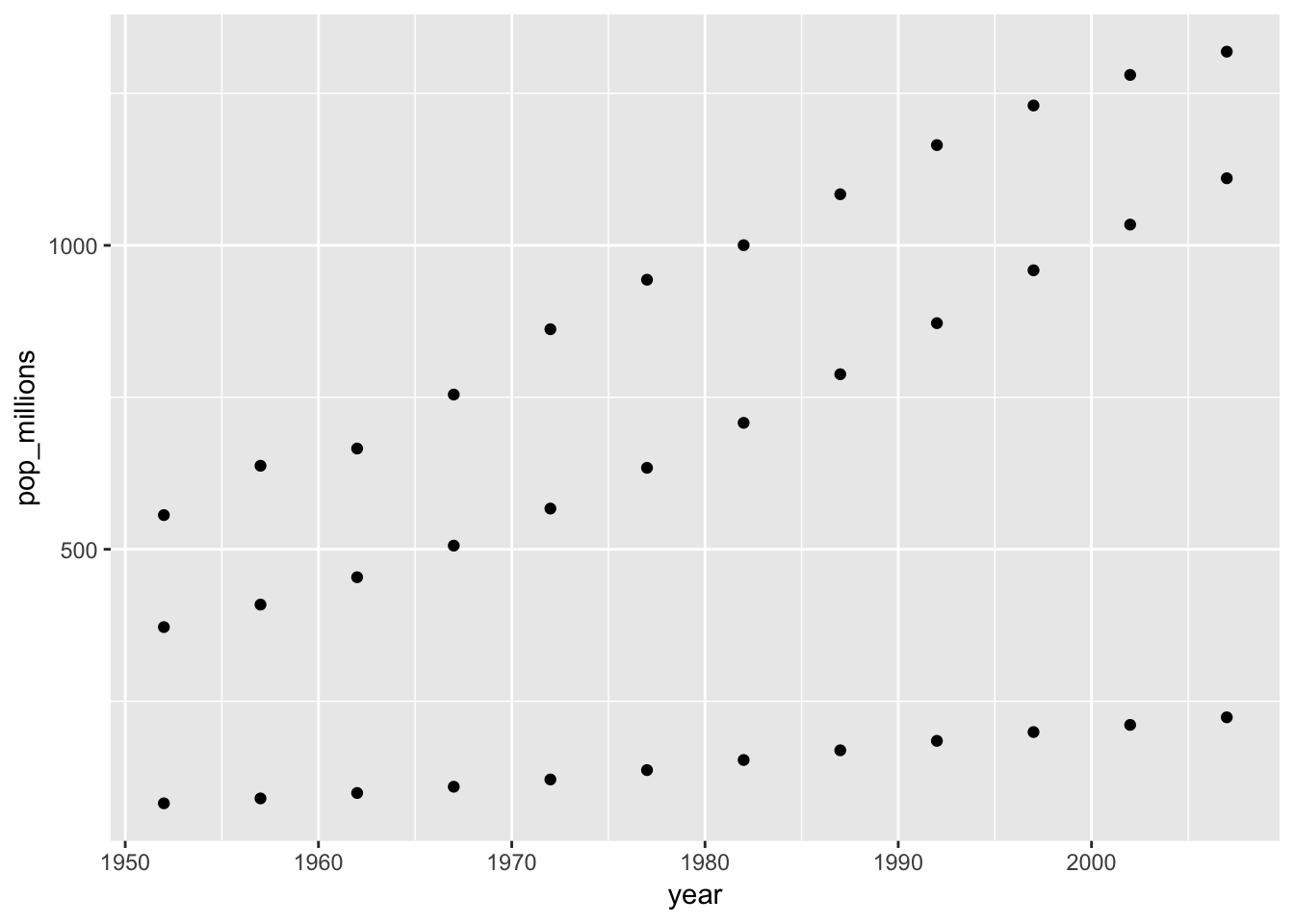# Vectorization

## Overview

Teaching: 10 min
Exercises: 15 min
Questions
• How can I operate on all the elements of a vector at once?

Objectives
• To understand vectorized operations in R.

Most of R’s functions are vectorized, meaning that the function will operate on all elements of a vector without needing to loop through and act on each element one at a time. This makes writing code more concise, easy to read, and less error prone.

``````x <- 1:4
x * 2
``````
``````##  2 4 6 8
``````

The multiplication happened to each element of the vector.

We can also add two vectors together:

``````y <- 6:9
x + y
``````
``````##   7  9 11 13
``````

Each element of `x` was added to its corresponding element of `y`:

``````x:  1  2  3  4
+  +  +  +
y:  6  7  8  9
---------------
7  9 11 13
``````

## Challenge 1

Let’s try this on the `pop` column of the `gapminder` dataset.

Make a new column in the `gapminder` data frame that contains population in units of millions of people. Check the head or tail of the data frame to make sure it worked.

## Solution to challenge 1

Let’s try this on the `pop` column of the `gapminder` dataset.

Make a new column in the `gapminder` data frame that contains population in units of millions of people. Check the head or tail of the data frame to make sure it worked.

``````gapminder\$pop_millions <- gapminder\$pop / 1e6
``````
``````##       country continent year lifeExp      pop gdpPercap pop_millions
## 1 Afghanistan      Asia 1952  28.801  8425333  779.4453     8.425333
## 2 Afghanistan      Asia 1957  30.332  9240934  820.8530     9.240934
## 3 Afghanistan      Asia 1962  31.997 10267083  853.1007    10.267083
## 4 Afghanistan      Asia 1967  34.020 11537966  836.1971    11.537966
## 5 Afghanistan      Asia 1972  36.088 13079460  739.9811    13.079460
## 6 Afghanistan      Asia 1977  38.438 14880372  786.1134    14.880372
``````

## Challenge 2

On a single graph, plot population, in millions, against year, for all countries. Don’t worry about identifying which country is which.

Repeat the exercise, graphing only for China, India, and Indonesia. Again, don’t worry about which is which.

## Solution to challenge 2

Refresh your plotting skills by plotting population in millions against year.

``````ggplot(gapminder, aes(x = year, y = pop_millions)) +
geom_point()
````````````countryset <- c("China","India","Indonesia")
ggplot(gapminder[gapminder\$country %in% countryset,],
aes(x = year, y = pop_millions)) +
geom_point()
``````Comparison operators, logical operators, and many functions are also vectorized:

Comparison operators

``````x > 2
``````
``````##  FALSE FALSE  TRUE  TRUE
``````

Logical operators

``````a <- x > 3  # or, for clarity, a <- (x > 3)
a
``````
``````##  FALSE FALSE FALSE  TRUE
``````

## Tip: some useful functions for logical vectors

`any()` will return `TRUE` if any element of a vector is `TRUE` `all()` will return `TRUE` if all elements of a vector are `TRUE`

Most functions also operate element-wise on vectors:

Functions

``````x <- 1:4
log(x)
``````
``````##  0.0000000 0.6931472 1.0986123 1.3862944
``````

Vectorized operations work element-wise on matrices:

``````m <- matrix(1:12, nrow=3, ncol=4)
m * -1
``````
``````##      [,1] [,2] [,3] [,4]
## [1,]   -1   -4   -7  -10
## [2,]   -2   -5   -8  -11
## [3,]   -3   -6   -9  -12
``````

## Tip: element-wise vs. matrix multiplication

Very important: the operator `*` gives you element-wise multiplication! To do matrix multiplication, we need to use the `%*%` operator:

``````m %*% matrix(1, nrow=4, ncol=1)
``````
``````##      [,1]
## [1,]   22
## [2,]   26
## [3,]   30
``````
``````matrix(1:4, nrow=1) %*% matrix(1:4, ncol=1)
``````
``````##      [,1]
## [1,]   30
``````

For more on matrix algebra, see the Quick-R reference guide

## Challenge 3

Given the following matrix:

``````m <- matrix(1:12, nrow=3, ncol=4)
m
``````
``````##      [,1] [,2] [,3] [,4]
## [1,]    1    4    7   10
## [2,]    2    5    8   11
## [3,]    3    6    9   12
``````

Write down what you think will happen when you run:

1. `m ^ -1`
2. `m * c(1, 0, -1)`
3. `m > c(0, 20)`
4. `m * c(1, 0, -1, 2)`

Did you get the output you expected? If not, ask a helper!

## Solution to challenge 3

Given the following matrix:

``````m <- matrix(1:12, nrow=3, ncol=4)
m
``````
``````##      [,1] [,2] [,3] [,4]
## [1,]    1    4    7   10
## [2,]    2    5    8   11
## [3,]    3    6    9   12
``````

Write down what you think will happen when you run:

1. `m ^ -1`
``````##           [,1]      [,2]      [,3]       [,4]
## [1,] 1.0000000 0.2500000 0.1428571 0.10000000
## [2,] 0.5000000 0.2000000 0.1250000 0.09090909
## [3,] 0.3333333 0.1666667 0.1111111 0.08333333
``````
1. `m * c(1, 0, -1)`
``````##      [,1] [,2] [,3] [,4]
## [1,]    1    4    7   10
## [2,]    0    0    0    0
## [3,]   -3   -6   -9  -12
``````
1. `m > c(0, 20)`
``````##       [,1]  [,2]  [,3]  [,4]
## [1,]  TRUE FALSE  TRUE FALSE
## [2,] FALSE  TRUE FALSE  TRUE
## [3,]  TRUE FALSE  TRUE FALSE
``````

## Challenge 4

We’re interested in looking at the sum of the following sequence of fractions:

`````` x = 1/(1^2) + 1/(2^2) + 1/(3^2) + ... + 1/(n^2)
``````

This would be tedious to type out, and impossible for high values of n. Use vectorisation to compute x when n=100. What is the sum when n=10,000?

## Challenge 4

We’re interested in looking at the sum of the following sequence of fractions:

`````` x = 1/(1^2) + 1/(2^2) + 1/(3^2) + ... + 1/(n^2)
``````

This would be tedious to type out, and impossible for high values of n. Can you use vectorisation to compute x, when n=100? How about when n=10,000?

``````sum(1/(1:100)^2)
``````
``````##  1.634984
``````
``````sum(1/(1:1e04)^2)
``````
``````##  1.644834
``````
``````n <- 10000
sum(1/(1:n)^2)
``````
``````##  1.644834
``````

We can also obtain the same results using a function:

``````inverse_sum_of_squares <- function(n) {
sum(1/(1:n)^2)
}
inverse_sum_of_squares(100)
``````
``````##  1.634984
``````
``````inverse_sum_of_squares(10000)
``````
``````##  1.644834
``````
``````n <- 10000
inverse_sum_of_squares(n)
``````
``````##  1.644834
``````

## Key Points

• Use vectorized operations instead of loops.